# Bihar Board Class 10th Maths Solutions Chapter 14 Statistics Ex 14.4

Bihar Board Class 10th Maths Solutions Chapter 14 Statistics Ex 14.4 Textbook Questions and Answers.

## BSEB Bihar Board Class 10th Maths Solutions Chapter 14 Statistics Ex 14.4Question 1.
The following distribution gives the daily income of 50 workers of a factory :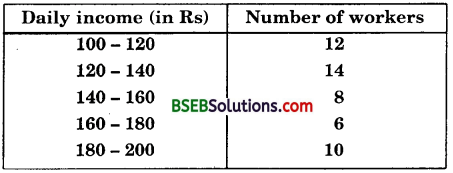Convert the distribution above to a less than type, cumulative frequency distribution and draw its ogive.
Solution:
Converting the given distribution to a less than type cumulative frequency distribution, we get:Let us now plot the points corresponding to the ordered pairs (120, 12), (140, 26), (160, 34), (180, 40) and (200, 50) on a graph.paper and join them by a free hand smooth curve.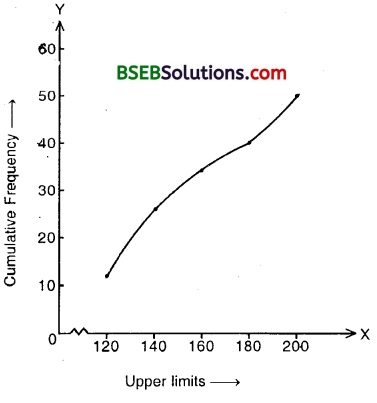The curve thus obtained is the required less than ogive.Question 2.
During the medical check-up of 35 students of a class, their weights were recorded as follows :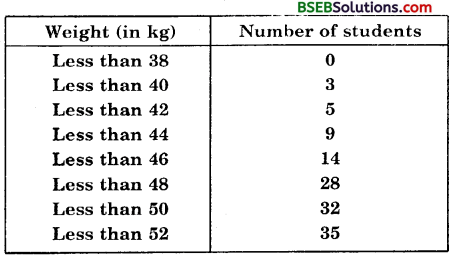Draw a less than type ogive for the given data. Hence obtain the median weight from the graph and verify the result by using the formula.
Solution:
Here the values 38, 40, 42, 44, 46, 48, 50 and 52 are the upper limits of the respective class-intervals. To represent the data in the table graphically, we mark the upper limits on the class intervals on x-axis and their corresponding cumulative frequencies on the y-axis, choosing a convenient scale.Let us now plot the points corresponding to the ordered pairs given by (38, 0), (40, 3), (42, 5), (44, 9), (46, 14), (48, 28), (50, 32) and (52, 35) on a graph paper and join them by a free hand smooth curve.
The curve thus obtained is the less than type ogive
To locate the median point on the graph, take $$\frac { n }{ 2 }$$ = $$\frac { 35 }{ 2 }$$ = 17.5 on the y-axis. From this point draw a line parallel to the x-axis cutting the curve at a. point. From this point, draw a perpendicular to the x-axis. The point of intersection of this perpendicular with the x-axis gives the median of the data. In this case it is 46.5.
Let us make the following table in order to find median by using the formula :Here, $$\frac { 35 }{ 2 }$$ , i.e., 17.5 will be in 46 – 48. Therefore 46 – 48 is the median class.
Here, l = 46, $$\frac { n }{ 2 }$$ = $$\frac { 35 }{ 2 }$$, cf = 14, f = 14
So, using the formula, median = l + $$\left(\frac{\frac{n}{2}-c f}{f}\right)$$ x h
Median = 46 + $$\frac { (17.5-14 }{ 14 }$$ x 2
= 46.5
Thus, median is verified.Question 3.
The following tables gives production yield per hectare of wheat of 100 farms of a village :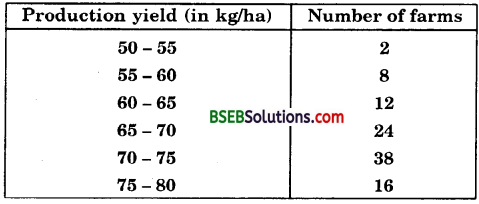Change the distribution to a more than type distribution, and draw its ogive.
Solution:
Converting the given distribution to a more than type distribution, we get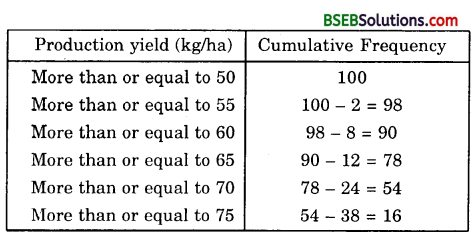Now, draw the ogive by plotting the points (50, 100), (55, 98), (60, 90), (65, 78), (70, 54) and (75, 16) on the graph paper and join them by a free hand smooth curve.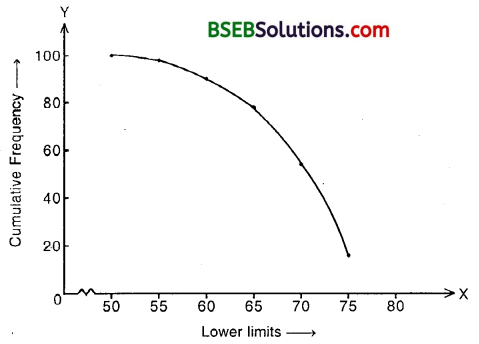The curve thus obtained is the required more than type ogive.